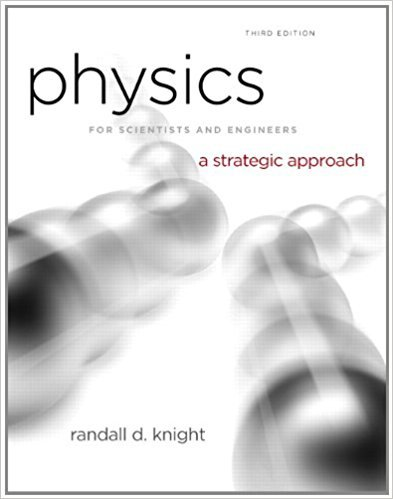×
Get Full Access to Physics For Scientists And Engineers: A Strategic Approach With Modern Physics - 3 Edition - Chapter 2 - Problem 35p
Get Full Access to Physics For Scientists And Engineers: A Strategic Approach With Modern Physics - 3 Edition - Chapter 2 - Problem 35p

×

# A ball rolls along the frictionless track shown in FIGURE.ISBN: 9780321740908 69

## Solution for problem 35P Chapter 2

Physics for Scientists and Engineers: A Strategic Approach with Modern Physics | 3rd Edition

• Textbook Solutions
• 2901 Step-by-step solutions solved by professors and subject experts
• Get 24/7 help from StudySoup virtual teaching assistantsPhysics for Scientists and Engineers: A Strategic Approach with Modern Physics | 3rd Edition

4 5 1 372 Reviews
20
5
Problem 35P

Problem 35P

A ball rolls along the frictionless track shown in FIGURE. Each segment of the track is straight, and the ball passes smoothly from one segment to the next without changing speed or leaving the track. Draw three vertically stacked graphs showing position, velocity, and acceleration versus time. Each graph should have the same time axis, and the proportions of the graph should be qualitatively correct. Assume that the ball has enough speed to reach the top.

FIGUREStep-by-Step Solution:

Solution 35P

Step 1 of 3

We are required to draw position-time, velocity-time and acceleration-time graphs for the given motion.

Step 2 of 3

Step 3 of 3

##### ISBN: 9780321740908

This full solution covers the following key subjects: ball, track, should, speed, segment. This expansive textbook survival guide covers 17 chapters, and 1439 solutions. Since the solution to 35P from 2 chapter was answered, more than 748 students have viewed the full step-by-step answer. The answer to “A ball rolls along the frictionless track shown in FIGURE. Each segment of the track is straight, and the ball passes smoothly from one segment to the next without changing speed or leaving the track. Draw three vertically stacked graphs showing position, velocity, and acceleration versus time. Each graph should have the same time axis, and the proportions of the graph should be qualitatively correct. Assume that the ball has enough speed to reach the top.FIGURE” is broken down into a number of easy to follow steps, and 76 words. The full step-by-step solution to problem: 35P from chapter: 2 was answered by , our top Physics solution expert on 08/30/17, 04:34AM. This textbook survival guide was created for the textbook: Physics for Scientists and Engineers: A Strategic Approach with Modern Physics, edition: 3. Physics for Scientists and Engineers: A Strategic Approach with Modern Physics was written by and is associated to the ISBN: 9780321740908.

Unlock Textbook Solution